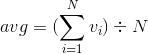# Semantics¶

## Primitive functions¶

A primitive is a built-in function for fundamental operations. There are various kinds of primitives in ELI.

• Basic arithmetic functions. `+ - * %`
• Functions for array manipulation.
• Object related functions. These function is eligible to operate on objects, such as lists and tables.

## User defined functions¶

This (UDF) allows programmers to define a function and use it afterwards. ELI inherits the design from APL which takes parameters from both left and right sides. A basic UDF is shown as follows.

```@.z<-foo x
z<-x+1
@.
```

The method `foo` takes one parameter `x` and saves `x+1` to `z` as returned value.

• If pass one parameter `x`, the function is `foo x`
• If pass two parameters `x` and `y`, the function is `y foo x`
• If pass zero parameter, the function is as simply as `foo`

Note

• The method `foo` should be declared in the script mood. Otherwise, the interpreter can’t recognize the method
• The execution order is always from right-to-left. So the argument on the right-hand side is evaluated first and the left-hand side follows

## Short functions¶

The format of short functions is `{foo:line0;...;lineN}` where `{foo}` is the function name and `{line0;...;lineN}` is the body of the function. There are some implicit rules defined as follows.

• If the literal `x` appears, it is the right argument of the function
• If the literal `y` appears, it is the left argument of the function
• If the literal `z` appears, it is the return value of the function
• Other names appear other than functions are localized as variables
• If the last line (lineN) is not empty, the result of the line is returned
• If the last line (lineN) is empty, nothing will be returned if `z` does not appear

A large program usually consists of many small functions which operate basic computation, such as `avg` (See the equation below) which returns the average of a vector or an array. For example, `avg !20` takes a vector consists of twenty numbers from 1 to 20. The expected result should be 10.5.The function `avg` can be defined in ELI interpreter quickly and easily as follows.

```      {avg: (+/x)%#x}
avg
avg !20
10.5
{avg}  //check code
---------avg--------
   z<-avg x
   z<-(+/x)%#x
--------------------
```

The short functions usually are defined for these functions with one line code. It provides more feasibility for fast programming to implement bright ideas. Ideally, it reduces the time cost to create a normal script files to load to active workspace.